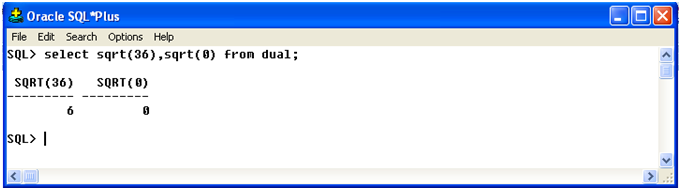## NUMBER FUNCTIONS in SQL

NUMBER FUNCTIONS :- Oracle has a variety of functions to process and manipulate number values in the database.These functions are also known as arithmatic functions,as these functions accept numeric input and return numeric values.
The various number functions are described below.

1-Command :- ABS
Purpose :-  It returns the absolute value of a number.
Example :-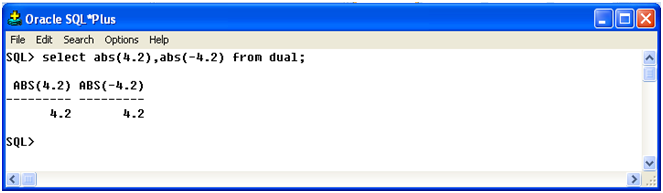2- Command :- LOG(m,n)
Purpose :-  It returns the logarithms base m of n,(m>1,n>0).
Example :-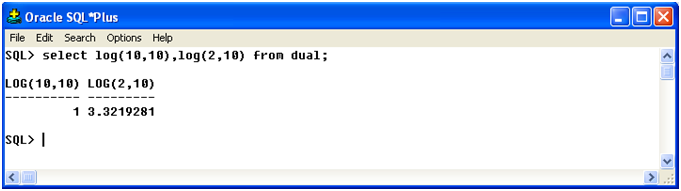3- Command :- MOD(m,n)
Purpose :-  It returns remainder of m divided by n.retuns m if n=0.
Example :-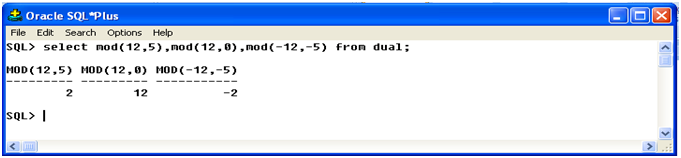4- Command :- ROUND(m,n)
Purpose :- It returns m rounded to n places right of the decimal point.
Example :-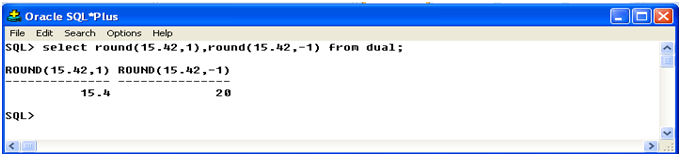5 - Command :- SIN
Purpose :- It returns the sin of a number.
Example :-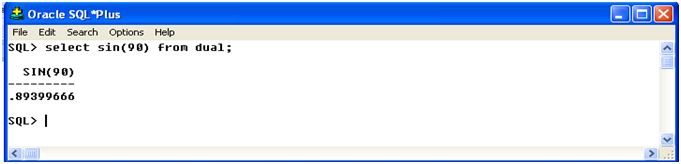6- Command :- COS
Purpose :-
It returns the cosent of a number.
Example :-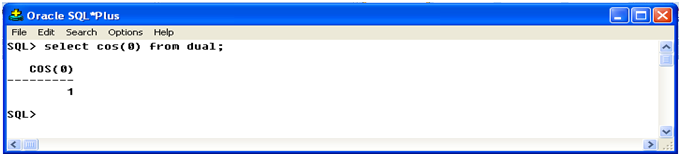7- Command :- TAN
Purpose :- It returns the tangent of a number.
Example :-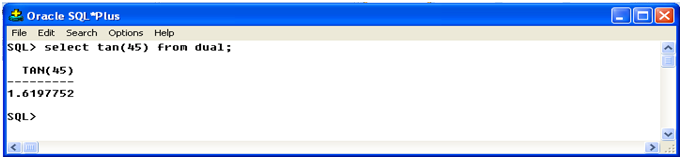8- Command :- TRUNC(m,n)
Purpose :-  It returns m truncated to n decimal places.
Example :-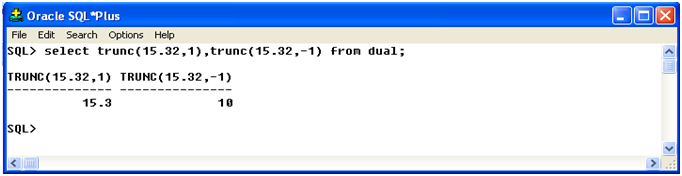9 - Command :- SQRT
Purpose :-  It returns the square root of a number.
Example :-